# Metric System - Definition with Examples

The Complete K-5 Math Learning Program Built for Your Child

• 40 Million Kids

Loved by kids and parent worldwide

• 50,000 Schools

Trusted by teachers across schools

• Comprehensive Curriculum

Aligned to Common Core

## Metric System Games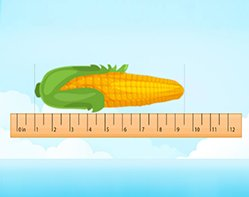Measuring Length - Customary Units

Use a inch ruler to measure the length of real world objects to the nearest inch and half inch.

Covers Common Core Curriculum 3.MD.4

## What is Metric System?

The metric system is a system of measurement that uses the meter, liter, and gram as base units of length (distance), capacity (volume), and weight (mass) respectively.

To measure smaller or larger quantities, we use units derived from the metric units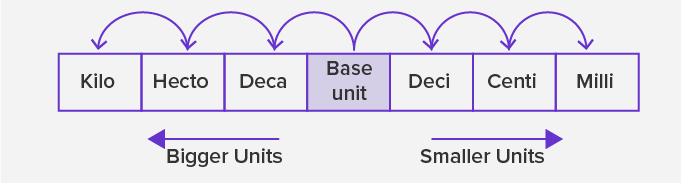• The given figure shows the arrangement of the metric units, which are smaller or bigger than the base unit.
• The units to the right of the base unit are smaller than the base unit. As we move to the right, each unit is 10 times smaller or one-tenth of the unit to its left. So, a ‘deci’ means one-tenth of the base unit, ‘centi’ is one-tenth of ‘deci’ or one-hundredth of the base unit and ‘milli’ is one-tenth of ‘centi’ or one-thousandth of the base unit.
• The units to the left of the base unit are bigger than the base unit. As we move to the left, each unit is 10 times greater than the unit to its right. So, a ‘deca’ means ten times of the base unit, ‘hecto’ is ten times of ‘deca’ or hundred times of the base unit and ‘killo’ is ten times of ‘hecto’ or thousand times of the base unit.

 Kilo Hecto Deca Base Unit Deci Centi Milli 1000 100 10 1 1/10 1/100 1/1000

So, the units for length, weight (mass) and capacity(volume) in the metric system are:

Length: Millimeter (mm), Decimeter (dm), Centimeter (cm), Meter (m), and Kilometer (km) are used to measure how long or wide or tall an object is.
Examples include measuring the thickness or length of debit card, length of cloth, or distance between two cities.

 Kilometer   (km) Hectometer   (hm) Decameter   (dam) Meter   (m) Decimeter   (dm) Centimeter   (cm) Millimeter   (mm) 1000 100 10 1 1/10 1/100 1/1000

Weight: Gram (g) and Kilogram(kg) are used to measure how heavy an object, using instruments.
Examples include measuring weight of fruits or, our own body weight.

 Kilogram   (kg) Hectogram   (hg) Decagram   (dag) Gram   (g) Decigram   (dg) Centigram   (cg) Milligram   (mg) 1000 100 10 1 1/10 1/100 1/1000

Capacity: Milliliter (ml) and Liter (l) are used to measure how much quantity of liquid an object can hold.
Examples include measuring the amount of juice in a juice can, or amount of water of in a water tank.

 Kiloliter   (kl) Hectoliter   (hl) Decaliter   (dal) Liter   (l) Deciliter   (dl) Centiliter   (cl) Milliliter   (ml) 1000 100 10 1 1/10 1/100 1/1000

Time: Second is the base unit for time. The other metric units of time are: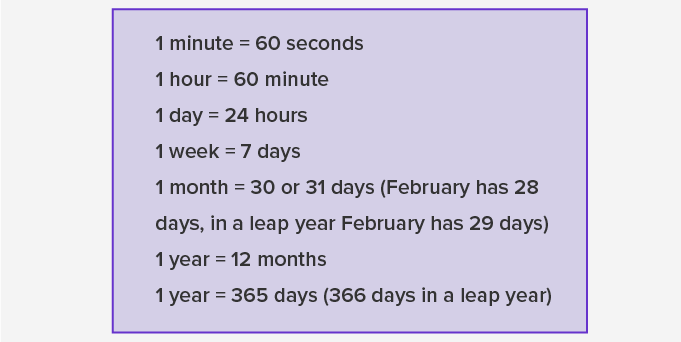Metric Conversions: Meters, grams and liters are considered the base units of length, weight and volume, respectively.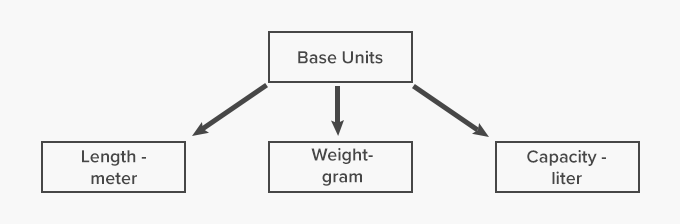Here’s how we can multiply or divide for making metric conversions. To convert a bigger unit to the smaller unit, we move left to write, we multiple by 10. Moving right to left, from smaller unit to bigger, we divide by 10.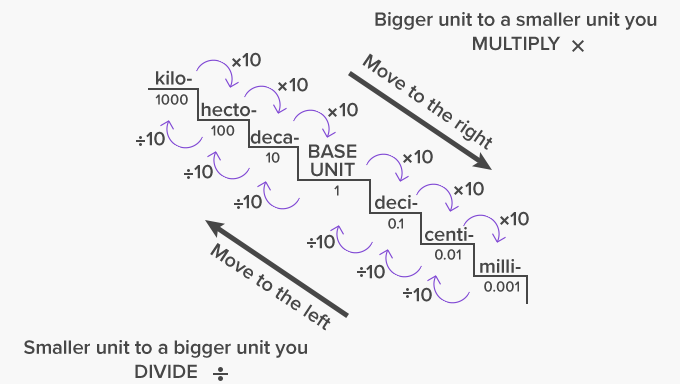Let us look at some examples of converting from one unit to another.

Example 1: Convert 5 km to m.

As 1 km = 1000 m

Therefore, 5 km = 5 × 1000 = 5000 m

Example 2: Convert 250 kg to milligrams.

We know, 1 g = 1000 mg and 1 kg = 1000 g

So, we first convert the kg to g as:

1 kg = 1000 g

Therefore, 250 kg = 250 × 1000 g = 250,000 g

Now, converting g to mg:

1 g = 1000 mg, therefore: 250,000 g = 250,000 × 1000 mg = 250,000,000 mg

Example 3: Convert 250 ml to liters.

1 liter = 1000 ml

Therefore, 450 ml = 450 ÷ 1000 = 0.45 liter

The US Standard Units or the Customary System uses customary units.

This system measures:

• Length or distance in inches, feet, yards, and miles.
• Capacity or volume in fluid ounces, cups, pints, quarts or gallons.
• Weight or mass in ounces, pounds and tons.

 Fun Facts A tonne is 1,000 kilograms. The metric system has its beginnings back 1670 by a mathematician called Gabriel Mouton.  Since the 1960s the metric system is called the "International System of Units" or "SI" (from the French "Système International"). The prefixes of the metric system can be remembered using the mnemonic “King Henry Died of Drinking Cold Milk”  There are units greater than the kilo as well. These have prefix mega, giga and tera. A mega is 1000 times of kilo. A giga is 1000 times of mega and a tera is 1000 times of a giga.

###Let's sing!

Find a millimeter in a pencil tip,
Find a centimeter in big paper clip.
Find a millilitre in the juice you drink,
Find a liter in the water filled in the sink.
Find a gram in the mango you ate,
Find a kilogram in it’s packet of eight!

###Let's do it!

Instead of handing our word problem worksheets to your child, site real life instances  in which the child is required to measure length, weight or capacity in metric units or convert bigger units into smaller units. For instance, at the grocery store, ask your child to weight 8 apples using a digital weight balance, in kilograms. Then ask him to convert the weight into grams and milligrams.

###Related math vocabulary

Won Numerous Awards & Honors